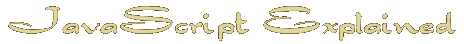Start | Time | IF | Date Object | Arrays | Avoid the work | Hide email | Random | Intigrate HTML | TestThe Date Object Explained

The Date Object is quite complex and holds a lot of data.
Here are some of the things it will do for you.

Once you have picked up the data from the computer
 now = new Date();
you can access all kinds of things
Normally you will be assigning a variable with a value but you can simply print out the answer on a page if you wish.
We already used the Year so with no further ado we could write the answer to a page like this.
 document.write( 'The year is now ' + now.getFullYear() )
You use this method to tell people what they already know

Or what they possibly don't know

I obviously used a tiny bit more than printing right on the page to get such things as day and month names but we will get into that later.

For reasons you will see later I will use variables to keep track and possibly change the data we get from the Date Object.
Here are the parameters I used to print out the above statements each set to its own variable

 Local Time h = now.getHours( ); The current hour (24 hour clock) mn = now.getMinutes( ); The minutes of the hour dy = now.getDay( ) ; The day in numbers Sunday = 0 to Saturday = 6 d = now.getDate( ) ; The day of the month 1 to 31 mth = now.getMonth( ); The month is in numbers January = 0 to December = 11 So let us fix that right away by adding 1 mth = now.getMonth( )+ 1 ; The month is now in numbers January = 1 to December = 12 yr = now.getFullYear( ) ; The year in four digits Universal Times (Greenwich Mean Time ) uh = now.getUTCHours( ); The current hour (24 hour clock) umn = now.getUTCMinutes( ); The minutes of the hour udy = now.getUTCDay( ) ; The day in numbers Sunday = 0 to Saturday = 6 ud = now.getUTCDate( ) ; The day of the month 1 to 31 umth = now.getUTCMonth( )+ 1 ; The month in numbers January = 1 to December = 12 uyr = now.getUTCFullYear( ) ; The year in four digits

From this you can see that we can print out the date and time in numbers like this.
 document.write( 'Date ' + dt + ' : ' + mth + ' : ' + yr + '
Time ' + h + ' : ' + mn )

If you don't like the 24 hour clock it is a simple matter to change it using a couple of 'if' statements.
In case we need the 24 hour clock we will make a new variable called ' hr ' equal to ' h ' and change it instead
If 'hr' is greater than 12 we need to subtract 12 from it or if it is 0 it should be 12.
 hr = h ; if (hr > 12 ) hr = hr - 12; if ( hr == 0 ) hr = 12;

Also the minutes if less than ten will not have a leading zero so another if statement will fix that but this would normally just be done in the print statement like this.
 document.write(' Time ' + hr + ' : ' ) if (mn<10)document.write('0' ) document.write(mn )

Next : Arrays

Home